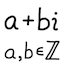#Factorize Gaussian integer (JavaScript)

## Program

 Number: Factorization:

## Description

This JavaScript program calculates the prime factorization of the given Gaussian integer. A Gaussian integer is a complex number of the form a + bi, where a and b are integers. This program has a limit of |a|, |b| < 226. If the number is large, the program may hang for a few seconds.

The factorization is put into the following canonical form:

• If the number is 0, 1, −1, i, or −i, then the factorization is the number itself.

• Otherwise the factorization begins with a factor of −1 or i or −i if necessary. The rest are primes listed in ascending order of absolute value, breaking ties by lowest argument (angle); each of these factors have absolute value > 1 and are in the first quadrant (0 ≤ argument < π/2).

The source TypeScript code and compiled JavaScript code are available for viewing. A Java version of the code is also available.

## Examples

• −1 = (−1) (unit)
• 2 = (−i) (1 + i) (1 + i)
• 4 + i = (4 + i) (prime)
• 7 = (7) (prime)
• 5 + 9i = (1 + i) (7 + 2i)

## Input format

• The real or imaginary component can be suppressed, and they can be in either order. The 1 can be suppressed for i. For example, all these are valid inputs: -0, 5i, 1+2i, -i-2

• Spaces are allowed anywhere in the Gaussian integer, except within a string of digits.

• To indicate negative numbers, the ASCII hyphen-minus (U+002D) and the minus sign (U+2212) are both acceptable. (The output properly uses the minus sign, though.)

• j is an acceptable synonym for i, for those electrical engineers out there.

## List of Gaussian primes

Table of all Gaussian primes up to norm 1000, with symmetries removed:

NumberNormArgument
1 + 1i245.00°
2 + 1i526.57°
3 + 0i90.00°
3 + 2i1333.69°
4 + 1i1714.04°
5 + 2i2921.80°
6 + 1i379.46°
5 + 4i4138.66°
7 + 0i490.00°
7 + 2i5315.95°
6 + 5i6139.81°
8 + 3i7320.56°
8 + 5i8932.01°
9 + 4i9723.96°
10 + 1i1015.71°
10 + 3i10916.70°
8 + 7i11341.19°
11 + 0i1210.00°
11 + 4i13719.98°
10 + 7i14934.99°
11 + 6i15728.61°
13 + 2i1738.75°
10 + 9i18141.99°
12 + 7i19330.26°
14 + 1i1974.09°
15 + 2i2297.59°
13 + 8i23331.61°
15 + 4i24114.93°
16 + 1i2573.58°
13 + 10i26937.57°
14 + 9i27732.74°
16 + 5i28117.35°
17 + 2i2936.71°
13 + 12i31342.71°
14 + 11i31738.16°
16 + 9i33729.36°
18 + 5i34915.52°
17 + 8i35325.20°
19 + 0i3610.00°
18 + 7i37321.25°
17 + 10i38930.47°
19 + 6i39717.53°
20 + 1i4012.86°
20 + 3i4098.53°
15 + 14i42143.03°
17 + 12i43335.22°
20 + 7i44919.29°
21 + 4i45710.78°
19 + 10i46127.76°
22 + 5i50912.80°
20 + 11i52128.81°
23 + 0i5290.00°
21 + 10i54125.46°
19 + 14i55736.38°
20 + 13i56933.02°
24 + 1i5772.39°
23 + 8i59319.18°
24 + 5i60111.77°
18 + 17i61343.36°
19 + 16i61740.10°
25 + 4i6419.09°
22 + 13i65330.58°
25 + 6i66113.50°
23 + 12i67327.55°
26 + 1i6772.20°
26 + 5i70110.89°
22 + 15i70934.29°
27 + 2i7334.24°
26 + 9i75719.09°
20 + 19i76143.53°
25 + 12i76925.64°
22 + 17i77337.69°
26 + 11i79722.93°
28 + 5i80910.12°
25 + 14i82129.25°
27 + 10i82920.32°
23 + 18i85338.05°
29 + 4i8577.85°
29 + 6i87711.69°
25 + 16i88132.62°
23 + 20i92941.01°
24 + 19i93738.37°
29 + 10i94119.03°
28 + 13i95324.90°
31 + 0i9610.00°
31 + 4i9777.35°
31 + 6i99710.95°

All the prime numbers in the table above are located in the first octant – a number has the form a + bi and satisfies a > 0, b ≥ 0, and ab (thus the argument is between 0° and 45°). They are listed in order of ascending norm, with ties broken by ascending argument. (“Norm” means squared absolute value.)

For each prime, a set of up to seven symmetrical primes have been excluded from the list. This is because if z is prime, then so are −z, iz, −iz, z (conjugate), −z, iz, and −iz. In terms of components, if z = a + bi is prime, then respectively so are −abi, −b + ai, bai, abi, −a + bi, b + ai, and −bai.

For example, 3 is prime, therefore so are −3, 3i, and −3i. For example, 5 + 2i is prime, therefore so are −5 − 2i, −2 + 5i, 2 − 5i, 5 − 2i, −5 + 2i, 2 + 5i, and −2 − 5i..